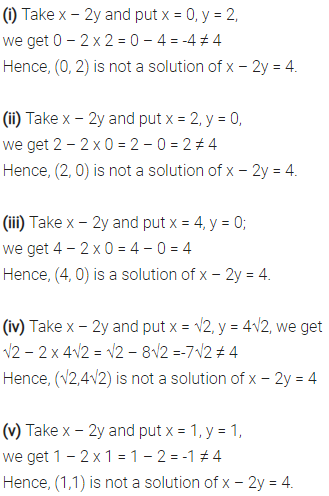# Check which of the following are solution of the equation x – 2y = 4

Check which of the following are solution of the equation x – 2y = 4 and which are not?
(i) (0, 2)
(ii) (2,0)
(iii) (4,0)
(iv) (√2,4√2)
(v) (1,1)